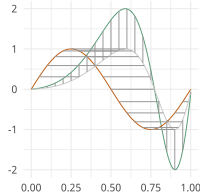# Functional Principal Component Analysis¶

Vertical and Horizontal Functional Principal Component Analysis using SRSF

moduleauthor:: J. Derek Tucker <jdtuck@sandia.gov>

class fPCA.fdahpca(fdawarp)[source]

This class provides horizontal fPCA using the SRVF framework

Usage: obj = fdahpca(warp_data)

Parameters: warp_data – fdawarp class with alignment data gam_pca – warping functions principal directions psi_pca – srvf principal directions latent – latent values U – eigenvectors coef – coefficients vec – shooting vectors mu – Karcher Mean tau – principal directions

Author : J. D. Tucker (JDT) <jdtuck AT sandia.gov> Date : 15-Mar-2018

calc_fpca(no=3, stds=array([-1, 0, 1]))[source]

This function calculates horizontal functional principal component analysis on aligned data

Parameters: no (int) – number of components to extract (default = 3) stds – number of standard deviations along gedoesic to compute (default = -1,0,1) fdahpca object of numpy ndarray srsf principal directions functional principal directions latent values coefficients eigenvectors
plot()[source]

plot plot elastic horizontal fPCA results

Usage: obj.plot()

class fPCA.fdajpca(fdawarp)[source]

This class provides joint fPCA using the SRVF framework

Usage: obj = fdajpca(warp_data)

Parameters: warp_data – fdawarp class with alignment data q_pca – srvf principal directions f_pca – f principal directions latent – latent values coef – principal coefficients id – point used for f(0) mqn – mean srvf U – eigenvectors mu_psi – mean psi mu_g – mean g C – scaling value stds – geodesic directions

Author : J. D. Tucker (JDT) <jdtuck AT sandia.gov> Date : 18-Mar-2018

calc_fpca(no=3, stds=array([-1., 0., 1.]), id=None, parallel=False, cores=-1)[source]

This function calculates joint functional principal component analysis on aligned data

Parameters: no (int) – number of components to extract (default = 3) id (int) – point to use for f(0) (default = midpoint) stds – number of standard deviations along gedoesic to compute (default = -1,0,1) parallel (bool) – run in parallel (default = F) cores (int) – number of cores for parallel (default = -1 (all)) fdajpca object of numpy ndarray srsf principal directions functional principal directions latent values coefficients eigenvectors
plot()[source]

plot plot elastic vertical fPCA result

Usage: obj.plot()

class fPCA.fdavpca(fdawarp)[source]

This class provides vertical fPCA using the SRVF framework

Usage: obj = fdavpca(warp_data)

Parameters: warp_data – fdawarp class with alignment data q_pca – srvf principal directions f_pca – f principal directions latent – latent values coef – principal coefficients id – point used for f(0) mqn – mean srvf U – eigenvectors stds – geodesic directions

Author : J. D. Tucker (JDT) <jdtuck AT sandia.gov> Date : 15-Mar-2018

calc_fpca(no=3, id=None, stds=array([-1, 0, 1]))[source]

This function calculates vertical functional principal component analysis on aligned data

Parameters: no (int) – number of components to extract (default = 3) id (int) – point to use for f(0) (default = midpoint) stds – number of standard deviations along gedoesic to compute (default = -1,0,1) fdavpca object containing srsf principal directions functional principal directions latent values coefficients eigenvectors
plot()[source]

plot plot elastic vertical fPCA result Usage: obj.plot()